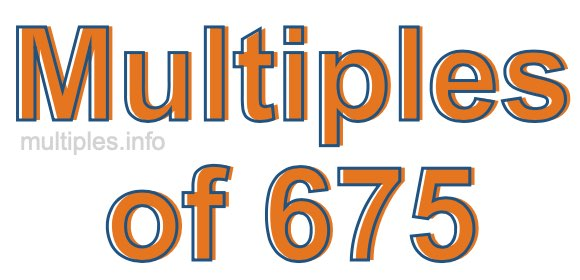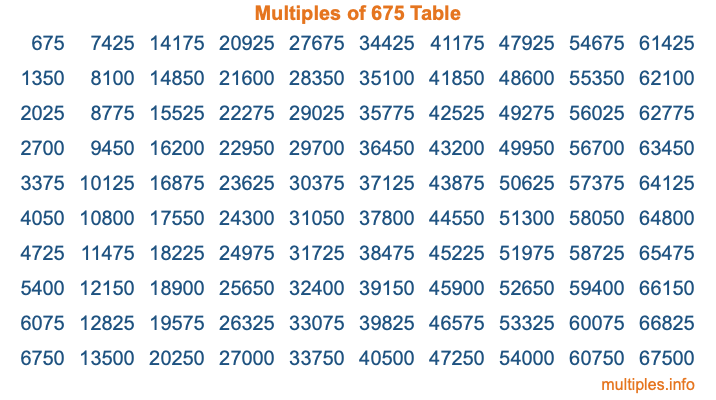Multiples of 675Welcome to the Multiples of 675 page. Here we will first teach you everything you will ever need to know about the multiples of 675, and then give you a study guide summary of everything we taught you to make sure you remember it all. Use this page to look up facts and learn information about the multiples of 675. This page will make you a multiples of six hundred seventy-five expert!

Definition of Multiples of 675
Multiples of 675 are all the numbers that when divided by 675 equal an integer. Each of the multiples of 675 are called a multiple. A multiple of 675 is created by multiplying 675 by an integer.

Therefore, to create a list of multiples of 675, you start with 1 multiplied by 675, then 2 multiplied by 675, then 3 multiplied by 675, and so on for as long as you want. Thus, the list of the first five multiples of 675 is 675, 1350, 2025, 2700, and 3375. To see a larger list of multiples of 675, see the printable image of Multiples of 675 further down on this page. We also have a category where you can choose any nth multiple of 675.

Multiples of 675 Checker
The Multiples of 675 Checker below checks to see if any number of your choice is a multiple of 675. In other words, it checks to see if there is any number (integer) that when multiplied by 675 will equal your number. To do that, we divide your number by 675. If the the quotient is an integer, then your number is a multiple of 675.

Is  a multiple of 675?

Least Common Multiple of 675 and ...
A Least Common Multiple (LCM) is the lowest multiple that two or more numbers have in common. This is also called the smallest common multiple or lowest common multiple and is useful to know when you are adding our subtracting fractions. Enter one or more numbers below (675 is already entered) to find the LCM.

Check out our LCM Calculator if you need more details about the Least Common Multiple or if you need the LCM for different numbers for adding and subtraction fractions.

nth Multiple of 675
As we stated above, 675 is the first multiple of 675, 1350 is the second multiple of 675, 2025 is the third multiple of 675, and so on. Enter a number below to find the nth multiple of 675.

th multiple of 675

Multiples of 675 vs Factors of 675
675 is a multiple of 675 and a factor of 675, but that is where the similarities end. All postive multiples of 675 are 675 or greater than 675. All positive factors of 675 are 675 or less than 675.

Below is the beginning list of multiples of 675 and the factors of 675 so you can compare:

Multiples of 675: 675, 1350, 2025, 2700, 3375, etc.

Factors of 675: 1, 3, 5, 9, 15, 25, 27, 45, 75, 135, 225, 675

As you can see, the multiples of 675 are all the numbers that you can divide by 675 to get a whole number. The factors of 675, on the other hand, are all the whole numbers that you can multiply by another whole number to get 675.

It's also interesting to note that if a number (x) is a factor of 675, then 675 will also be a multiple of that number (x).

Multiples of 675 vs Divisors of 675
The divisors of 675 are all the integers that 675 can be divided by evenly. Below is a list of the divisors of 675.

Divisors of 675: 1, 3, 5, 9, 15, 25, 27, 45, 75, 135, 225, 675

The interesting thing to note here is that if you take any multiple of 675 and divide it by a divisor of 675, you will see that the quotient is an integer.

Multiples of 675 Table
Below is an image of the first 100 multiples of 675 in a table. The table is in chronological order, column by column. The first column has the first ten multiples of 675, the second column has the next ten multiples of 675, and so on.The Multiples of 675 Table is also referred to as the 675 Times Table or Times Table of 675. You are welcome to print out our table for your studies.

Negative Multiples of 675
Although not often discussed or needed in math, it is worth mentioning that you can make a list of negative multiples of 675 by multiplying 675 by -1, then by -2, then by -3, and so on, to get the following list of negative multiples of 675:

-675, -1350, -2025, -2700, -3375, etc.

Multiples of 675 Summary
Below is a summary of important Multiples of 675 facts that we have discussed on this page. To retain the knowledge on this page, we recommend that you read through the summary and explain to yourself or a study partner why they hold true.

There are an infinite number of multiples of 675.

A multiple of 675 divided by 675 will equal a whole number.

675 divided by a factor of 675 equals a divisor of 675.

The nth multiple of 675 is n times 675.

The largest factor of 675 is equal to the first positive multiple of 675.

675 is a multiple of every factor of 675.

675 is a multiple of 675.

A multiple of 675 divided by a divisor of 675 equals an integer.

675 divided by a divisor of 675 equals a factor of 675.

Any integer times 675 will equal a multiple of 675.

Multiples of a Number
Here you can get the multiples of another number, all with the same attention to detail as we did for multiples of 675 on this page.

Multiples of
Multiples of 676
Did you find our page about multiples of six hundred seventy-five educational? Do you want more knowledge? Check out the multiples of the next number on our list!